In focus

# Basic Functions Of SQL Server 2014

## In this article we will discuss the functions of SQL Server 2014.

Anmol Garg Mar 11, 2016

SQL Server provides many built -in and user-defined functions. Some of the functions are discussed below:

Square() : The Square function is given to return the square of the given expression and returns the float value in SQL Server 2014.

Syntax : SQUARE (float_expression)

Example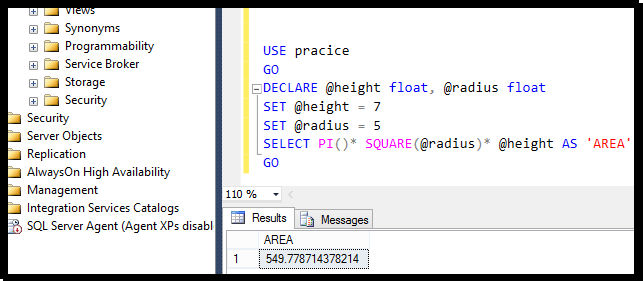SQRT (float_number) : The Sqrt functions are used to returns a square root of a floating-point number. The SQL SQRT function accepts only positive arguments and returns an error when a negative argument is passed in SQL Server 2014.

Syntax
: SQRT(<numeric_expression>)

Example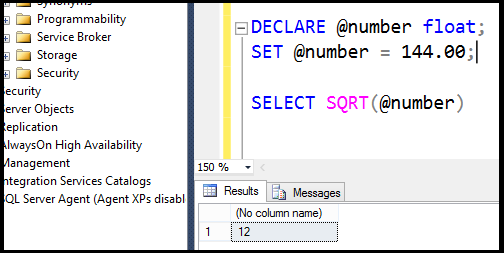ATN()2 :  An ATN2 () function is a mathematical function that returns the angle in radians between the positive x-axis and the ray from the origin to the point (y, x), x and y are the values of the two specified float expressions in SQL. It returns a double-precision number between -1 and 1.

Syntax : ATN2 (float_expression , float_expression)

It takes two arguments, both are the float_expression, an expression of the type float or of a type that can be implicitly converted to float. It has a return type float only.

Example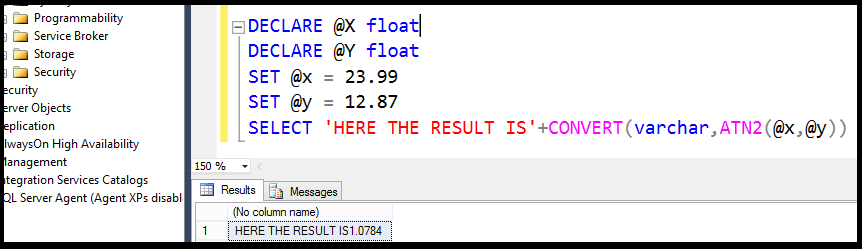EOMONTH() :  The EOMONTH () is used to get the last day of the month that contains the specified date with an optional offset. This function can be remote to the SQL Server 2014 servers and higher, but cannot be remote to the servers with a version lower than SQL Server 2014.

Syntax : EOMONTH ( start_date [ month_to_add ] )

It takes two arguments.

First is the Start_date, the date expression specifying the date for which to return the last day of the month.

Second, the month_to_add, an Optional integer expression specifying the number of months to add to the start_date. It has the return type, date only.

Example 1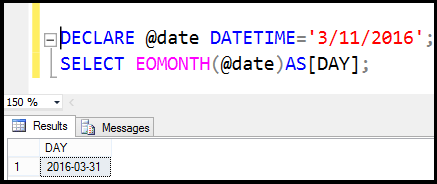Example 2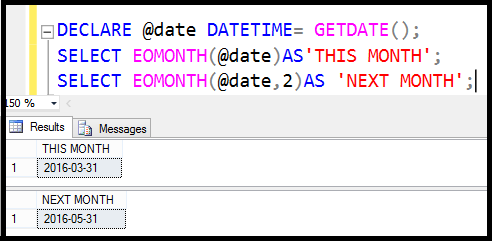LEN() :  The length of a string means the number of characters or symbols in a string. Hence, to get the length of a string we can use the LEN() function in SQL Server 2014.

Syntax : int LEN(String)

This function takes one argument as the string and returns the number of characters in the string.

Example 1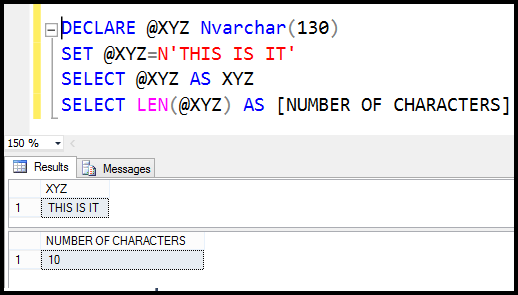Number of characters in SQL Server 2014.

Here there are 2 outputs, output 1 is simply counting the characters, while output 2 is also counting the blank spaces between characters.

Example 2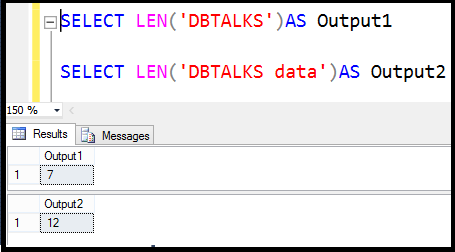@@ NEST LEVEL()
:   The @@NESTLEVEL () function is a configuration function in SQL Server 2014. It is used to get the nesting level of the current stored procedure execution (initially 0) on the local server.

Syntax : @@NESTLEVEL

It has return type int only. We can use the @@NESTLEVEL() function as shown.

Example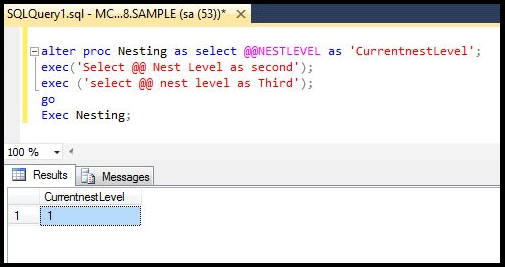DAY() :  The DAY() is used to get an integer representing the day (day of the month) of the specified date.

Syntax : DAY ( date )

It takes only one argument date, which is an expression that can be resolved to a time, date, smalldatetime, datetime, datetime 2, or a datetimeoffset value. It has a return type int only. We can use the DAY() function as given.

Example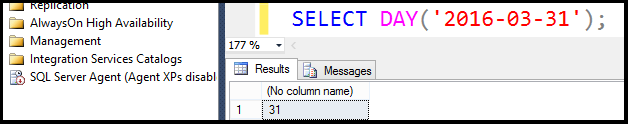CAST()
:   The Cast() functions are used to convert a data type variable or a data from one data type to another data type. The Cast () function provides a data type of a dynamic parameter (?) or a NULL value.

Syntax : CAST ([Expression] AS Datatype)

The data type to which you are casting an expression is the target type. The data type of the expression from which you are casting, is the source type in SQL.

Example 1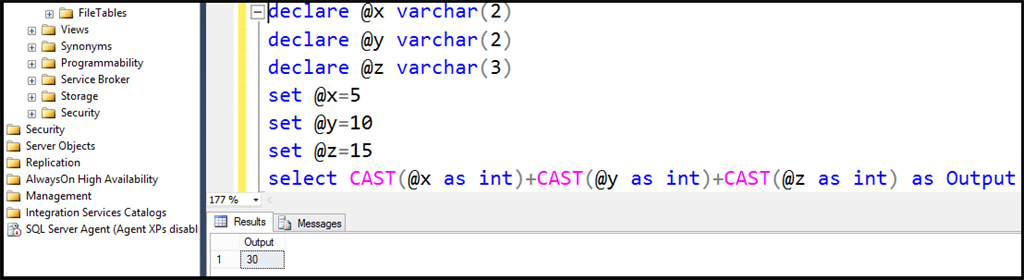Example 2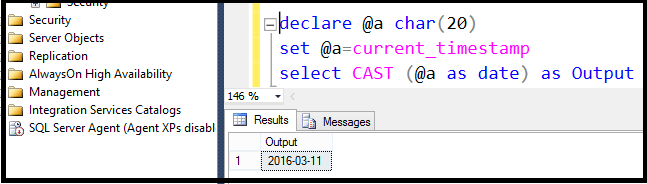Here's a free e-book on SQL Server: Introducing Microsoft SQL Server 2014

sql server